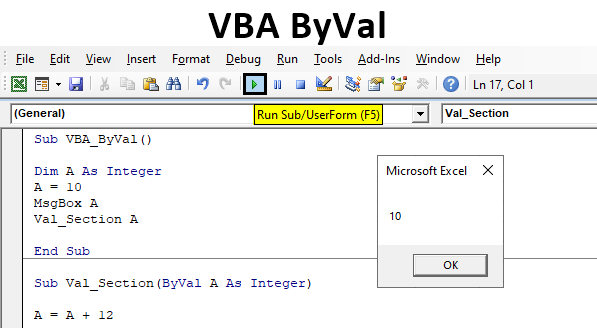# How To Use Excel VBA Macros - Byref And Byval

Oct 15, 2020 • edited Oct 18, 2020You can pass arguments to a procedure (function or sub) by reference or by value. By default, Excel VBA passes arguments by reference. As always, we will use an easy example to make things more clear.

Place a command button on your worksheet and add the following code lines:

Dim x As Integer

x = 10

MsgBox Triple(x)

MsgBox x

The code calls the function Triple. It's the result of the second MsgBox we are interested in. Functions need to be placed into a module.

1. Open the Visual Basic Editor and click Insert, Module.

2. Add the following code lines:

Function Triple(ByRef x As Integer) As Integer

x = x * 3

Triple = x

End Function

Result when you click the command button on the sheet:3. Replace ByRef with ByVal.

Function Triple(ByVal x As Integer) As Integer

x = x * 3

Triple = x

End Function

Result when you click the command button on the sheet:Explanation: When passing arguments by reference we are referencing the original value. The value of x (the original value) is changed in the function. As a result the second MsgBox displays a value of 30. When passing arguments by value we are passing a copy to the function. The original value is not changed. As a result the second MsgBox displays a value of 10 (the original value).

#Tutorial#How To#VBA#Function and Sub

How to Create Excel Array formulas and functions

How to use the WEEKDAY Function (VBA) In Excel